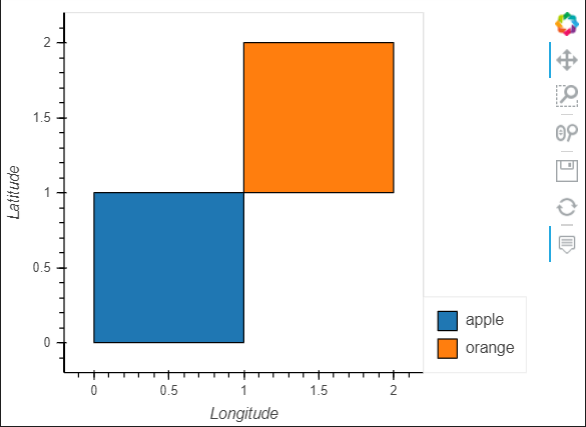# Apply a colormap to LineStrings

When plotting polygons in a geodataframe with hvplot, you can easily apply a colormap based on an attribute value (numeric or categorical):

`polygon_df.hvplot(geo=True, color='category')`I’d expect the same to apply to polylines:

`polylines_df.hvplot(geo=True, color='category')`

However, that raises the following error and an empty plot.

``````BokehUserWarning: ColumnDataSource's columns must be of the same length.
Current lengths: ('category', 0), ('color', 2), ('xs', 2), ('ys', 2)
``````

Is this functionality not supported for polylines? Or is there some different syntax? I would think plotting continuous or discrete values as a colormap on polylines should be possible. Thanks!

Please see the hopefully reproducible example below:

``````# %%
import numpy as np
import geopandas as gpd
import hvplot.pandas
from shapely.geometry import LineString, Polygon

#%% Create Sample Coordinates
coords = np.array(((0., 0.), (0., 1.), (1., 1.), (1., 0.), (0., 0.)))

#%% Create Polygons and add to geodataframe with sample data
pg1 = Polygon(coords)
pg2 = Polygon(coords + 1)

polygon_df = gpd.GeoDataFrame({'geometry': [pg1, pg2],
'value':[0 , 1],
'category': ['apple', 'orange']},
geometry='geometry')
# %% Plot successfully with hvplot
polygon_df.hvplot(geo=True, color='category')

# %% Create Linestrings and add to geodataframe with sample data
ls1 = LineString(coords[:2])
ls2 = LineString(coords[2:4])

linestring_df = gpd.GeoDataFrame({'geometry': [ls1, ls2],
'value':[0 , 1],
'category': ['apple', 'orange']},
geometry='geometry')

#%% Plot lines successfully with no color
linestring_df.hvplot(geo=True)

# %% Plot unsuccessfully (Blank Plot and BokehUserWarning) when coloring by value or category
linestring_df.hvplot(geo=True, color='category')

# BokehUserWarning: ColumnDataSource's columns must be of the same length.
# Current lengths: ('category', 0), ('color', 2), ('xs', 2), ('ys', 2)
``````

I think this is somewhat similar to an unresolved question from @jsanjayce although I am not using datashader.

1 Like